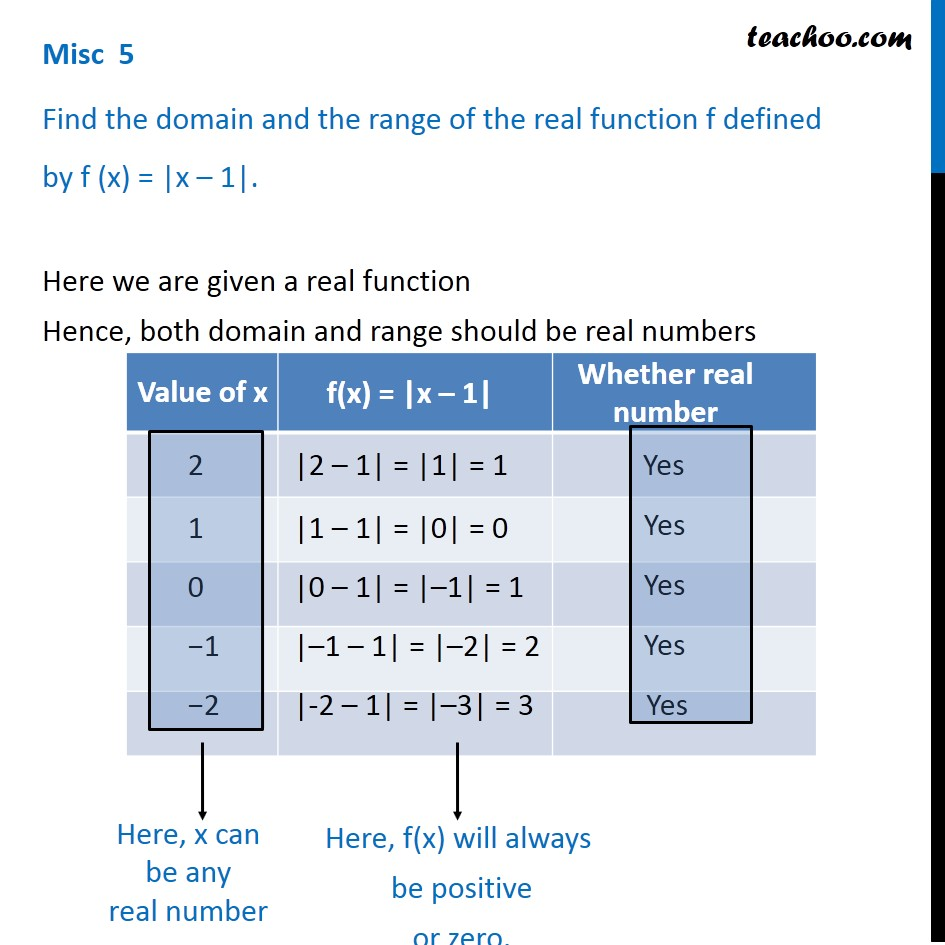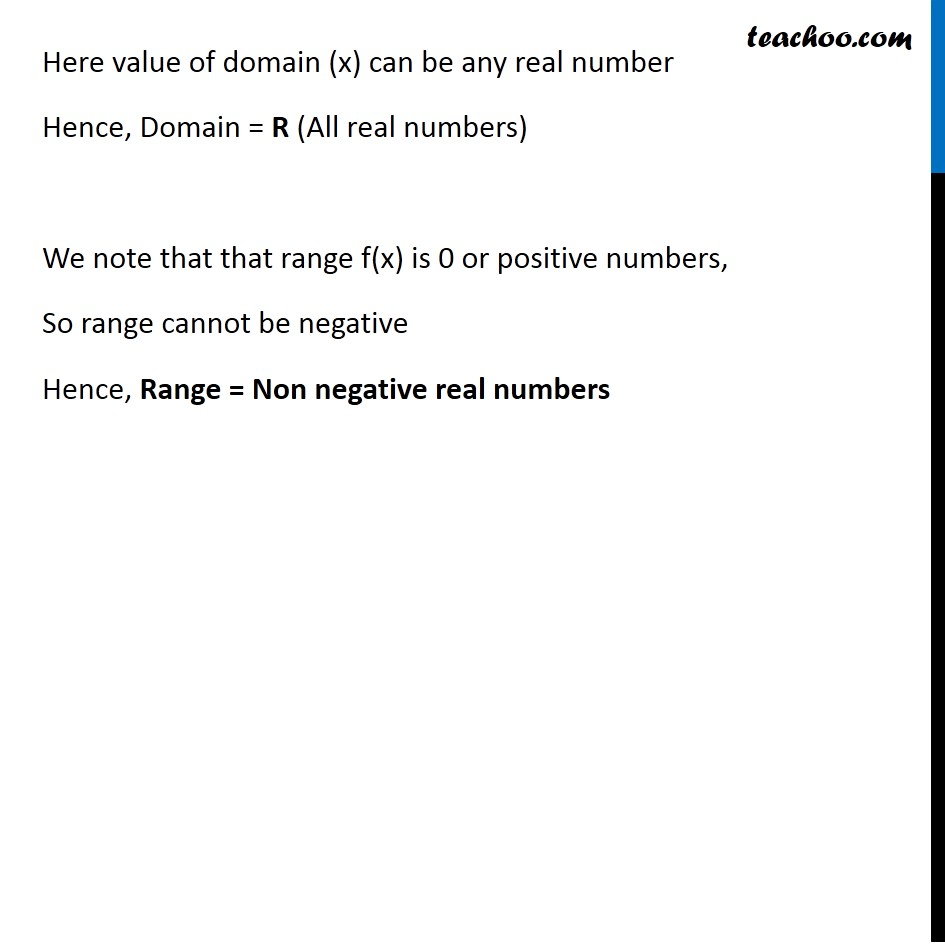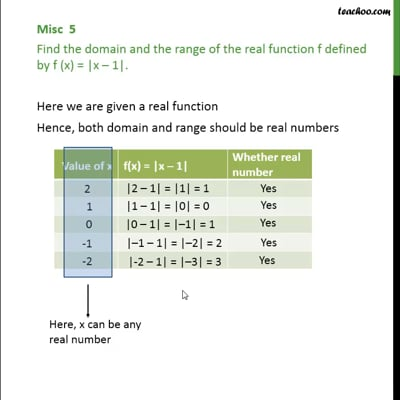Miscellaneous

Chapter 2 Class 11 Relations and Functions
Serial order wiseThis video is only available for Teachoo black users

Learn in your speed, with individual attention - Teachoo Maths 1-on-1 Class

### Transcript

Misc 5 Find the domain and the range of the real function f defined by f (x) = |x – 1|. Here we are given a real function Hence, both domain and range should be real numbers Here, x can be any real number Here, f(x) will always be positive or zero. Here value of domain (x) can be any real number Hence, Domain = R (All real numbers) We note that that range f(x) is 0 or positive numbers, So range cannot be negative Hence, Range = Non negative real numbers